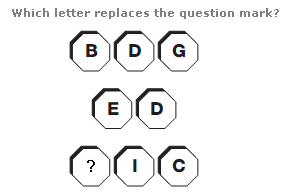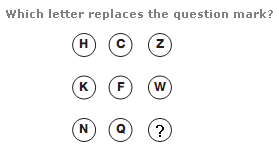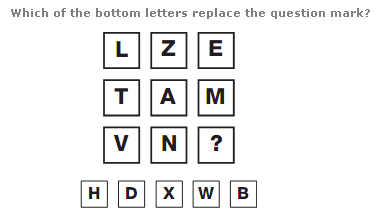# Missing letters puzzles interview questions

Answer : AExplanation : Converting each letter to its numerical value, the diagram represents a subtraction sum: 247 – 54 = 193.
Answer : TAnswer : W